# Convert Excel Time to Unix Time in Excel & Google Sheets

This tutorial will demonstrate how to convert a time in Excel’s format to Unix time in Excel and Google Sheets.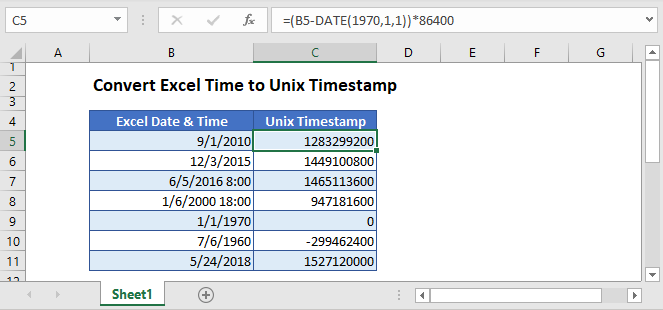## What is Unix Time?

Unix time is also known as Epoch Time or POSIX time or Unix timestamp. It’s a system that counts the number of seconds that have elapsed since the Unix Epoch, i.e. January 1st, 1970. To put in simple words, Unix time is the total number of seconds between a date and Unix Epoch.

## Convert Excel Time to Unix Time

To calculate Unix time, first, we need to find the total number of days between the Excel time and Epoch time. Then multiply the calculated days by 86,400, because a day has 86,400 seconds (24 hours × 60 minutes ×60 seconds = 86,400 seconds).

``=(B5-DATE(1970,1,1))*86400``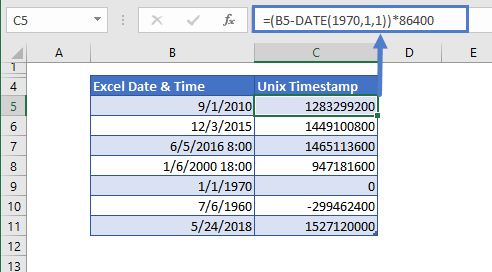### Step by Step Explanation

The first step in the conversion of Excel time to Unix time is to calculate the numeric value of the Epoch date. This can be done by using the DATE function.

``=DATE(1970,1,1)``

It gives us this value:

=25569

In the same way, the calculation of the numeric value of Epoch date is subtracted from the given Excel time’s numeric value

``=(B5-DATE(1970,1,1))``

=(40422-25569)
=(14853)

After that, the difference calculated from above is multiplied by 86400 to get the resultant value in seconds

=(14853)*86400

This is the total number of seconds, we get, between two dates

=1283299200

## Convert Unix Time to Excel Time

Inversely, we can convert the Unix time to Excel time with the help of the following formula:

``````=(B3/86400)+DATE(1970,1,1)
``````

###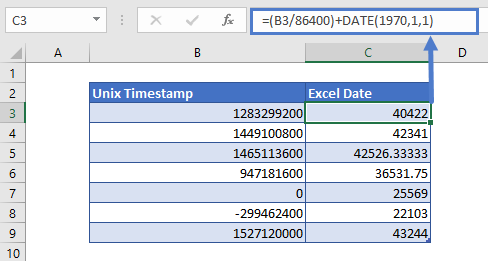Step By Step Explanation

First, the Unix timestamp is divided by the total number of seconds in a day, i.e. 86400.

``=B3/86400``

=14853

Now, we calculate the numeric value of the Epoch date through DATE function

``=DATE(1970,1,1)``

=25569

Once we have the numeric value of the Epoch date, we’ll add these two values

= 14853 + 25569
= 40422

The resultant value, we got after the calculation, is in the serial date number format. To view it in date format, simply change the format of the cell to Date or your required custom format from the Number Format by accessing it through the Home Tab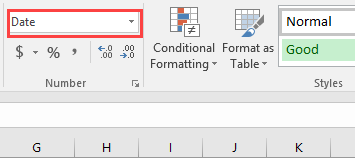or by pressing CTRL + 1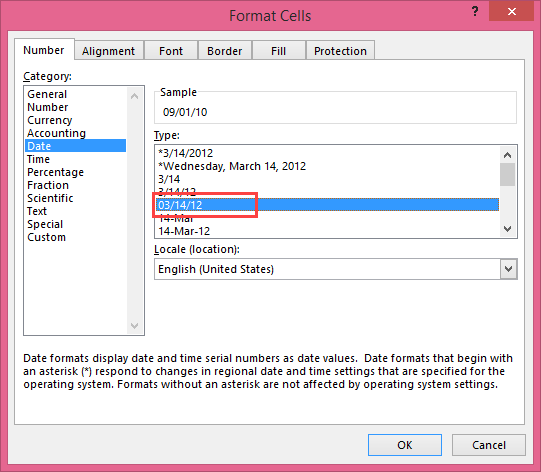After changing the format of the numeric values of the Excel date & time, we get the following Excel date and time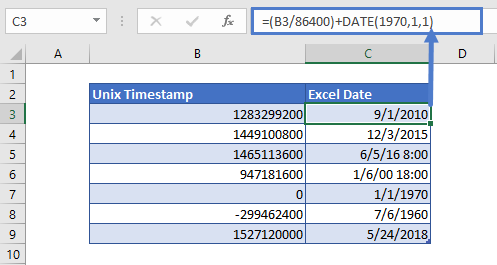## Convert Excel Time to Unix Time in Google Sheets

The conversion formula for time to Unix time works exactly the same in Google Sheets as in Excel.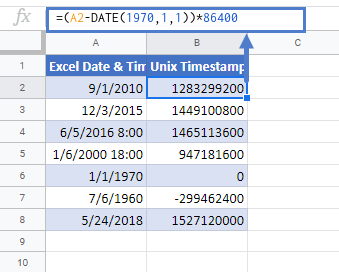### Excel Practice Worksheet

Practice Excel functions and formulas with our 100% free practice worksheets!